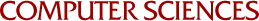## Estimating the Accuracy of Quasi Monte Carlo Integration

John H. Halton
1971

The author discusses the doubtful value of error-bounds and estimates of a statistical nature, based on variance-estimators and the Central Limit Theorem, when used in situations where quasirandom (deterministic) sets of points are used to estimate integrals over multi-dimensional intervals. (The doubt extends, of course, to all quasi-random calculations.) He describes an alternative approach, based on discrepancies of point-sets and consequent bounds on the error, for integrands of bounded variation in the sense of Hardy and Krause. Suitable error-estimates, computable during the calculation of the main estimator, are described.